JEE  >  Test: Ray Optics & Optical Instruments - 3

# Test: Ray Optics & Optical Instruments - 3

Test Description

## 26 Questions MCQ Test Physics Class 12 | Test: Ray Optics & Optical Instruments - 3

Test: Ray Optics & Optical Instruments - 3 for JEE 2023 is part of Physics Class 12 preparation. The Test: Ray Optics & Optical Instruments - 3 questions and answers have been prepared according to the JEE exam syllabus.The Test: Ray Optics & Optical Instruments - 3 MCQs are made for JEE 2023 Exam. Find important definitions, questions, notes, meanings, examples, exercises, MCQs and online tests for Test: Ray Optics & Optical Instruments - 3 below.
Solutions of Test: Ray Optics & Optical Instruments - 3 questions in English are available as part of our Physics Class 12 for JEE & Test: Ray Optics & Optical Instruments - 3 solutions in Hindi for Physics Class 12 course. Download more important topics, notes, lectures and mock test series for JEE Exam by signing up for free. Attempt Test: Ray Optics & Optical Instruments - 3 | 26 questions in 50 minutes | Mock test for JEE preparation | Free important questions MCQ to study Physics Class 12 for JEE Exam | Download free PDF with solutions
 1 Crore+ students have signed up on EduRev. Have you?
Test: Ray Optics & Optical Instruments - 3 - Question 1

### When a plane mirror AB is placed horizontally on level ground at a distance of 60 metres from the foot of a tower, the top of the tower and its image in the mirror subtends, an angle of 90° at B. The height of the tower is: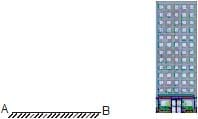Detailed Solution for Test: Ray Optics & Optical Instruments - 3 - Question 1

The angle subtended at the eye by the top of the tower with respect to the ground is 45 degrees. This happens when the height of the tower is equal to the distance from the eye. The eye is assumed to be very near to the mirror. (Refer below figure).
Angle subtended by AB = 90 degrees/2 = 45 degrees.
Therefore, tanθ=h/60​. That is, tan45=h/60​.So, h=60m.
Hence, the height of the tower is 60 m.Test: Ray Optics & Optical Instruments - 3 - Question 2

### A unnumbered wall clock shows time 04 : 25 : 37, where 1st term represents hours, 2nd represents minutes & the last term represents seconds, What time will its image in a plane mirror show.

Detailed Solution for Test: Ray Optics & Optical Instruments - 3 - Question 2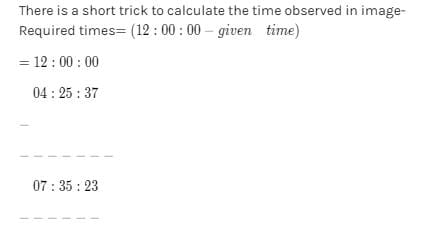Test: Ray Optics & Optical Instruments - 3 - Question 3

### Two plane mirrors of length L are separated by distance L and a man M2 is standing at distance L from the connecting line of mirrors as shown in figure. A man M1 is walking in a straight line at distance 2L parallel to mirrors at speed u, then man M2 at O will be able to see image of M1 for total time :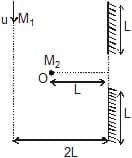Detailed Solution for Test: Ray Optics & Optical Instruments - 3 - Question 3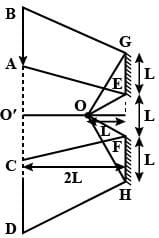The light from man M1​ hits the mirror and then reaches the man M2​. The positions of the moving man need to be found when the light from him hits the corners of the mirror to reach the standing man.
From geometry,
AO′=L/2​+2(L/2​)
=3L/2
​Similarly, BO′=(3L/2​)+2(3L/2​)
=9L/2
Hence AB=(9L/2)-(3L/2)=3/2
Time taken to cover this distance=3L/u​
Similarly time taken to cover CD=3L/u​
Thus total time he is visible is 6L/u​

Test: Ray Optics & Optical Instruments - 3 - Question 4

A person is standing in a room of width 200 cm. A plane mirror of vertical length 10 cm is fixed on a wall in front of the person. The person looks into the mirror from distance 50 cm. How much width (height) of the wall behind him will he be able to see : (assume that he uses the full mirror)

Detailed Solution for Test: Ray Optics & Optical Instruments - 3 - Question 4

Width of the room = 200cm (Given)
Length of plane mirror = 10cm  (Given)
Distance of the person looking into mirror = 50cm  (Given)
In first triangle -
TanФ = x/200 --- 1
In second triangle -
TanФ = 5/50 --- 2
Equating both the equations -
5/50 = x/200
x = 20cm
Thus, the height of the wall is 2x+10 = 50
The width of the wall he will be able to see = 50cm

Test: Ray Optics & Optical Instruments - 3 - Question 5

Variation of focal length to form a sharp image on retina is called

Detailed Solution for Test: Ray Optics & Optical Instruments - 3 - Question 5

The ability of the eye in terms of variation of the focal length to form sharp images on the retina is called accommodation.

In simpler terms, the ability of the eye to change the focus from a near distance or a far distance is called accommodation of the eye.
It is possible because of the lens which changes its shape. This makes it possible for the eye to keep focus on an object even when its distance from the eye changes.

Test: Ray Optics & Optical Instruments - 3 - Question 6

Which of the following cannot form real image of a real object ?

Detailed Solution for Test: Ray Optics & Optical Instruments - 3 - Question 6

A concave mirror can form both real and virtual images depending upon the distance between object and mirror.

A convex mirror can only form virtual, erect, and diminished images; therefore, it is true that it can never form a real image of a real object.

-Virtual image cannot be formed from a virtual object.
- When an object is placed between pole and focus, the image formed by the concave mirror is magnified, virtual and erect.
-When an object is placed beyond the centre of curvature , image of it is formed between centre of curvature and focus which is diminished,real and inverted,so real image of real object can be formed by concave mirror.
-When the object is virtual, the image formed is real for the concave surface as shown in figure.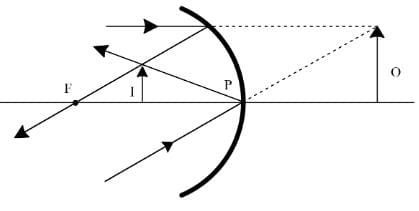*Multiple options can be correct
Test: Ray Optics & Optical Instruments - 3 - Question 7

The radius of curvature of the left & right surface of the concave lens are 10 cm & 15 cm respectively. The radius of curvature of the mirror is 15 cm.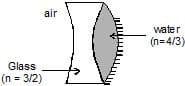Detailed Solution for Test: Ray Optics & Optical Instruments - 3 - Question 7

Peq=2PL1+2PL2+PM
=(2/fL1)+2(1/fL2)-(1/fM)
Peq=-2(1/12)+(4/45)-(2/-15)
Peq=1/18
-1/f=1/18
F=-18cm
Here the system acts as a concave mirror.

*Multiple options can be correct
Test: Ray Optics & Optical Instruments - 3 - Question 8

If a symmetrical biconcave thin lens is cut into two identical halves. They are placed in different ways as shown :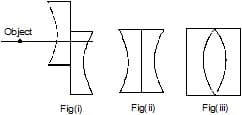Detailed Solution for Test: Ray Optics & Optical Instruments - 3 - Question 8

There form 3 images due to refraction on two different lenses 3 times one upper ,1 refraction from both lenses, 1 at the bottom.
now focus of Plano concave =f=R/(1−μ)
hence focus for diagram ii> f=R/2(1−μ)
and same focus for diagram iii>because again there is combination of two two Plano concave lens
so, the ratio of focus is 1:1

*Multiple options can be correct
Test: Ray Optics & Optical Instruments - 3 - Question 9

A convex lens forms an image of an object on screen. The height of the image is 9 cm. The lens is now displaced until an image is again obtained on the screen. The height of this image is 4 cm. The distance between the object and the screen is 90 cm.

Detailed Solution for Test: Ray Optics & Optical Instruments - 3 - Question 9

hobject2​=himage1​×himage2
hobject​=√36​=6cm
magnification of image is v/u​=9/6​
v=3u​/2
in lens displacement method, u+v=d; uv=df
u+(3u/2)​=90
u=36   => v=54cm
uv=df
f=(36×54)/90​=21.6

*Multiple options can be correct
Test: Ray Optics & Optical Instruments - 3 - Question 10

A narrow beam of white light goes through a slab having parallel faces

Detailed Solution for Test: Ray Optics & Optical Instruments - 3 - Question 10

Refraction of light of different wavelength is shown in the figure.The light inside the slab clearly splits up into different colors. The emergent ray from a single white ray is a beam of different colors, parallel to the incident ray. However the emergent beam is white, because the white rays adjacent to the incident white ray above also split into different colors that add up to these wavelengths to produce a white beam of light parallel to the incident white beam.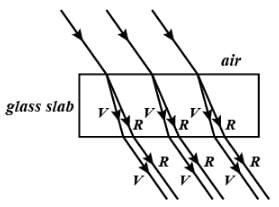*Multiple options can be correct
Test: Ray Optics & Optical Instruments - 3 - Question 11

By properly combining two prisms made of different materials, it is possible to

Detailed Solution for Test: Ray Optics & Optical Instruments - 3 - Question 11

We can either have deviation or not and same for dispersion as only μ is different and angle of prism is of our choice
option A,B,C are correct.

Test: Ray Optics & Optical Instruments - 3 - Question 12

A transparent sphere of radius R and refractive index m. An object O is placed at a distance x from the pole of the first surface so that a real image is formed at the pole of the exactly opposite surface.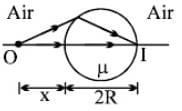If x = 2R, then the value of m is

Detailed Solution for Test: Ray Optics & Optical Instruments - 3 - Question 12

(μ/v)- (μ/u) = (μ2- μ1)/R
Or, (μ/2R) + (1/2R) = μ-1/R
Or, (μ/2) + (1/2) = (μ-1)/1
Or, (Μ+1)/2= μ-1
Or, μ+1=2μ-2
μ=3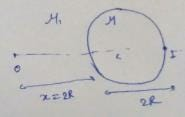Test: Ray Optics & Optical Instruments - 3 - Question 13

A transparent sphere of radius R and refractive index m. An object O is placed at a distance x from the pole of the first surface so that a real image is formed at the pole of the exactly opposite surface.If x = ∞, then the value of m is

Detailed Solution for Test: Ray Optics & Optical Instruments - 3 - Question 13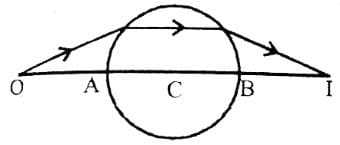From the symmetry of the fig.ray inside the sphere is parallel to the principal axis. Taking refraction at A
2/v)−(μ1/u))=(μ2−μ1)/R
(μ/∞)−(1/−R)=(μ−1)/R
(1/R)=(μ−1)/R⇒μ−1=1
μ=2

Test: Ray Optics & Optical Instruments - 3 - Question 14

A transparent sphere of radius R and refractive index m. An object O is placed at a distance x from the pole of the first surface so that a real image is formed at the pole of the exactly opposite surface.If an object is placed at a distance R from the pole of first surface, then the real image is formed at a distance R from the pole of the second surface. The refractive index m of the sphere is given by

Detailed Solution for Test: Ray Optics & Optical Instruments - 3 - Question 14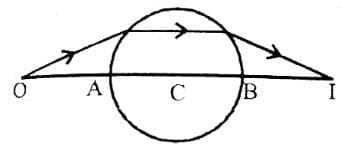From the symmetry of the fig.ray inside the sphere is parallel to the principal axis. Taking refraction at A
2/v)−(μ1/u))=(μ2−μ1)/R
(μ/∞)−(1/−R)=(μ−1)/R
(1/R)=(μ−1)/R⇒μ−1=1
μ=2

Test: Ray Optics & Optical Instruments - 3 - Question 15

Column -II shows the optical phenomenon that can be associated with optical components given in column-I. Note that column-I may have more than one matching options in column-II.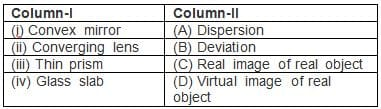Test: Ray Optics & Optical Instruments - 3 - Question 16

Statement-I : If a source of light is placed in front of rough wall its image is not seen.

Statement-II : The wall does not reflect light.

Test: Ray Optics & Optical Instruments - 3 - Question 17

Statement-I : As the distance x of a parallel ray from axis increases, focal length decreases

Statement-II : As x increases, the distance from pole to the point of intersection of reflected ray with principal axis decreases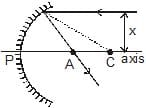Test: Ray Optics & Optical Instruments - 3 - Question 18

Statement-I : When an object dipped in a liquid is viewed normally, the distance between the image and the object is indepedent of the height of the liquid above the object.

Statement-II : The normal shift is independent of the location of the slab between the object and the observer.

Detailed Solution for Test: Ray Optics & Optical Instruments - 3 - Question 18

a) At first the height of the liquid level is obviously dependent because the normal shift will happen according to the depth of the liquid.
Drawing a ray diagram keeping a coin at very deep and another close to the surface.We will notice that the earlier one will have a noticeable change of position but the later one will only change a bit.
b) The position of the slab is independent because wherever we keep it the incident ray & the emergent ray at last will be parallel (same medium keeping in mind).

Test: Ray Optics & Optical Instruments - 3 - Question 19

Statement-I : When two plane mirrors are kept perpendicular to each other as shown ( O si the point object), 3 image will be formed.

Statement-II : In case of multiple reflection, image of one surface can act as an object for the next surface.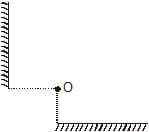Test: Ray Optics & Optical Instruments - 3 - Question 20

Statement-I : A piece of paper placed at the position of a real image of a virtual object of intense light will burn after sufficient time.

Statement-II : A virtual object is that point where the incident rays appear to converge and a real image is that point at which reflected/ refracted rays actually converge.

*Answer can only contain numeric values
Test: Ray Optics & Optical Instruments - 3 - Question 21

Find the angle of deviation (clockwise) suffered by a ray incident on a plane mirror, at an angle of incidence 30°.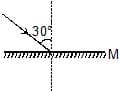Detailed Solution for Test: Ray Optics & Optical Instruments - 3 - Question 21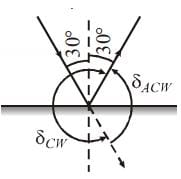δ ACW = 180° – 60°
= 120° Anticlockwise
δ CW = 180° + 60°
= 240° Clockwise

*Answer can only contain numeric values
Test: Ray Optics & Optical Instruments - 3 - Question 22

Find the angle of deviation (anticlockwise) suffered by a ray incident on a plane mirror, at an angle of incidence 30°.Detailed Solution for Test: Ray Optics & Optical Instruments - 3 - Question 22

δ ACW = 180° – 60°
= 120° Anticlockwise
δ CW = 180° + 60°
= 240° Clockwise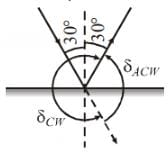*Answer can only contain numeric values
Test: Ray Optics & Optical Instruments - 3 - Question 23

Figure shows a plane mirror onto which a light ray is incident. If the incident light ray is turned by 10° and the mirror by 20°, as shown, find the angle turned by the reflected ray.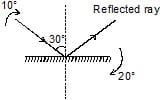Detailed Solution for Test: Ray Optics & Optical Instruments - 3 - Question 23

The reflected ray will turn 100 due to incident ray and 400 due to mirror in opposite direction
∴ Net rotation =40−10
=300
Hence,

*Answer can only contain numeric values
Test: Ray Optics & Optical Instruments - 3 - Question 24

A light ray is incident on a plane mirror, which after getting reflected strikes another plane mirror, as shown in figure. The angle between the two mirrors is 60°. Find the angle 'q' shown in figure.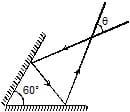Detailed Solution for Test: Ray Optics & Optical Instruments - 3 - Question 24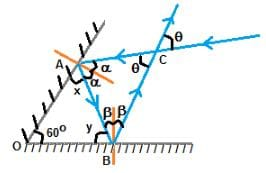Figure shows two plane mirrors kept at angle 60°.  A light ray incidents at A making an angle of incidence α,
reflected and again incidents at B making an angle β and getting reflected .
Reflected ray from B makes angle θ with the initial incident ray.
At reflection point A, as shown in figure, we have α+x = 90° ..............(1)
At reflection point B, as shown in figure, we have β+y = 90° ..............(2)
By adding (1) and (2), after substituting x+y = 120°, we get,  α+β = 60° ...............(3)
From ΔABC, we have,  2α + 2β + θ = 180° ...................(4)
Hence By using eqn.(3), we get , θ = 180 - 120 = 60°

Test: Ray Optics & Optical Instruments - 3 - Question 25

Find focal length of mirror that forms an image 6.2 cm behind mirror of an object placed at 26 cm in front of mirror?

Detailed Solution for Test: Ray Optics & Optical Instruments - 3 - Question 25

Here, given that,
v=-6.2 and u=26
we have to find,f=?
So, we know,
1/f=(1/u)+(1/v)
1/f=(1/-6.2) + (1/26)
f=-8.41cm
Hence the correct answer is option B.

*Answer can only contain numeric values
Test: Ray Optics & Optical Instruments - 3 - Question 26

There are two plane mirror inclined at 40°, as shown. A ray of light is incident on mirror M1. What should be the value of angle of incidence 'i' so that the light ray retraces its path after striking the mirror M2.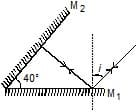Detailed Solution for Test: Ray Optics & Optical Instruments - 3 - Question 26

The ray strikes M2 normally
so r=90o-40o=50o
to the horizontal
=40 to the normal
As i=r
i=40o

## Physics Class 12

157 videos|452 docs|213 tests
 Use Code STAYHOME200 and get INR 200 additional OFF Use Coupon Code
Information about Test: Ray Optics & Optical Instruments - 3 Page
In this test you can find the Exam questions for Test: Ray Optics & Optical Instruments - 3 solved & explained in the simplest way possible. Besides giving Questions and answers for Test: Ray Optics & Optical Instruments - 3, EduRev gives you an ample number of Online tests for practice

## Physics Class 12

157 videos|452 docs|213 tests